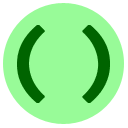# 15A: Termination Determination

Exercises 15A, 15B, and 15C can be completed in any order.

In this lesson you will complete a long and complex problem that has been broken into parts for you. This lesson also introduces `str.split()`, a useful method for splitting strings: it deletes all of the spaces, and then returns a list of all of the words in the string. The original string is not modified.

Example
Examples of `str.split()`You can call `split()` with special arguments to do more sophisticated splitting, but we don't need this ability below. If you are interested, check the Python documentation.

Now we get to the task at hand. The old programming language BASIC was famous for its numbered lines and `goto` statements. For this exercise, you will implement a simple version of BASIC with just these features. Specifically, the input to your program will consist of several lines in the format

`«label» goto «target»`

where `«label»` and `«target»` are positive integers. The label is like the name or address of the line; all labels are unique. The target tells you the label of the line to move to next. The last line of the program is `«label» END` indicating that you should stop once you move to this line. Here is a sample BASIC program:

```5 GOTO 30
10 GOTO 20
20 GOTO 10
30 GOTO 40
40 END```
When BASIC runs the program, this is what happens. We begin at the first line (with label 5). The line with label 5 has target 30, so next we go to the line with label 30. Then line 30 tells us to go to line 40. Line 40 tells us to END. So, the program terminated successfully.

On the other hand, a BASIC program can also loop forever. Here is an example:

```10 GOTO 21
21 GOTO 37
37 GOTO 21
40 END```
The program starts at line 10, but then it loops forever between lines 21 and 37.

Your task is to write a Python program, which reads a BASIC program as input. If the program terminates, your program should print `success`. If the program enters an infinite loop, your program should print `infinite loop`. Assume each target equals some valid label and that all labels are unique, so you do not have to do error-checking.

There are several ways you could do this, but in this lesson we have chosen one simple approach that breaks the problem in to 3 sub-tasks. (In lesson 15C you have one large problem and design the sub-tasks yourself.)

To read the program, we need to keep calling `input()`. However, we need to stop calling `input()` once the last line (the one with `END`) is reached, to avoid an `EOFError`.Write a function `getBASIC()` which takes no arguments, and does the following: it should keep reading lines from input using a while loop; when it reaches the end it should return the whole program in the form of a list of strings. (Hints: about lists and stopping)
You may enter input for the program in the box below.

## Sub-task 2: Go to it!

Once we have read the program, we need to be able to move from line to line in the program. To accomplish this, we ask you to write the following subroutine.Coding Exercise: Go to it!
Define a function `findLine(prog, target)` to perform the following. Assume `prog` is a list of strings containing a BASIC program, like the type generated by `getBASIC()`; assume `target` is a string containing a line number, which is the target of a GOTO statement. The function should return the index `i` (a number between `0` and `len(prog)-1`) such that `prog[i]` is the line whose label equals `target`. Hint
Sample input/output: If you call

`findLine(['10 GOTO 20','20 END'], '10')`
then the output should be `0`, since item 0 of the list is the line with label 10.
Enter testing statements like `print(myfunction("test argument"))` below.

In the previous two exercises, we handled the input routine and the searching task. These will be useful to make writing the main program shorter. However, there is still a major question: how can we actually solve the original problem? The most straightforward way would be to simulate the BASIC program:

• let `prog` be the BASIC program (an array of strings)
• start a counter called `location` at 0, since we begin on the first line of the program
• while True,
• if `prog[location]` is the END line, return "success" and stop.
• let `T` be the target string indicated in `prog[location]`
• let the new value of `location` be `findLine(prog, T)`

But, there is a major problem: this doesn't detect infinite loops, and if the BASIC program has an infinite loop, then the Python program will also loop forever. What we wanted was that the program should print "infinite loop" in this situation. We leave this problem for you to solve; we give a hint below.Coding Exercise: Smart Simulation
Write a function `execute(prog)` to do the following. Assume `prog` is a list of strings containing the BASIC program, like before. Then, your function should return either "`success`" or "`infinite loop`" depending on whether the program terminates, or loops forever. Important: you should assume the procedure `findLine(prog, target)` defined in sub-task 2 is already defined, you do not need to re-write it. Hint
Enter testing statements like `print(myfunction("test argument"))` below.

## Putting it all together

To test your code as a complete solution, copy and paste your previous solutions into the following template.Coding Exercise: BASIC SimulatorIf your programming language is slightly more complicated, then it's impossible to write a termination-checking program. This was the earliest important theorem in computer science, proven by Alan Turing in the 1930s, and nowadays people refer to the result by saying "the Halting Problem is undecidable."